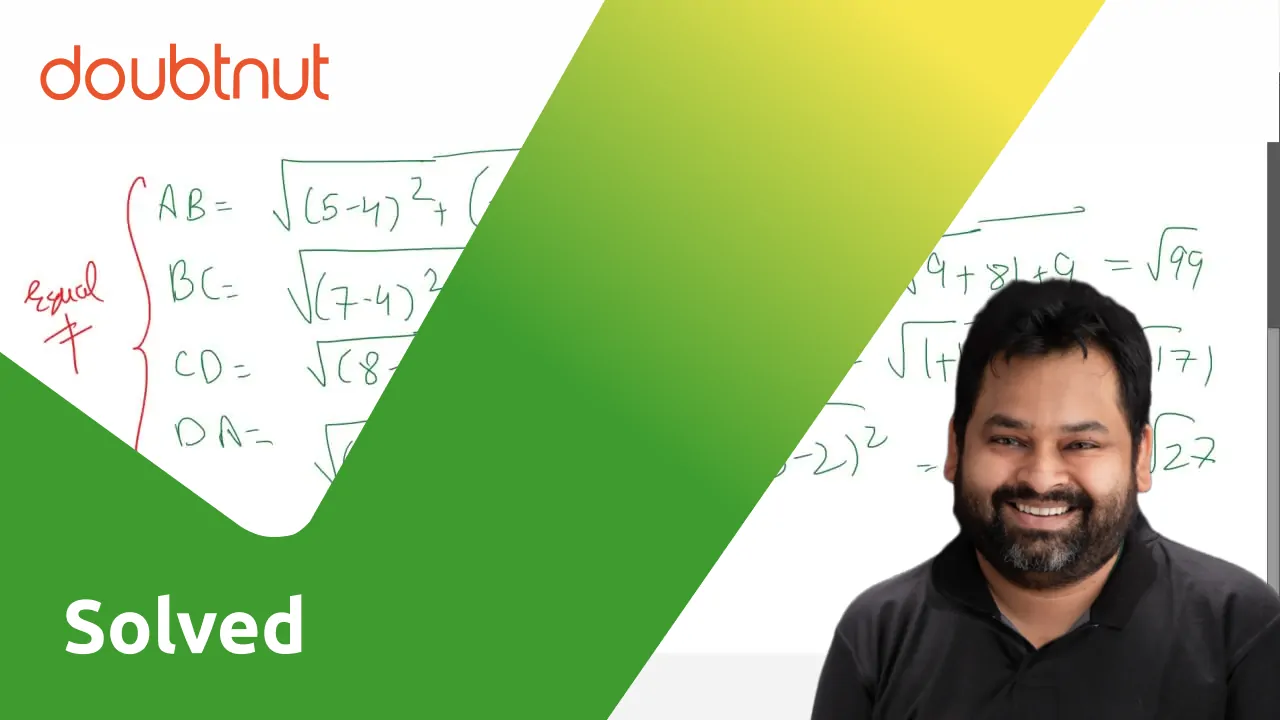Doubtnut App - Get 50 Lakh+ video solutions better than GoogleInstall Now

# The points (5,-4,2), (4,-3,1), (7,6,4) and (8,-7,5) are the vertices of a. a rectangle                  b.  a square                c. a parallelogram           d. none of these

Text Solution
Verified by Experts

## Let, A(5,−4,2),B(4,−3,1),C(7,6,4)andD(8,−7,5) So, AB=√(5−4)2+(−4+3)2+(2−1)2=√1+1+1=√3 BC=√(4−7)2+(−3−6)2+(1−4)2=√9+81+9=√99=3√11 CD=√(7−8)2+(6+7)2+(4−5)2=√1+169+1=√171 DA=√(8−5)2+(−7+4)2+(5−2)2=√9+9+9=√27=3√3 As, we see none of the sides are equal then option (D) is correct.